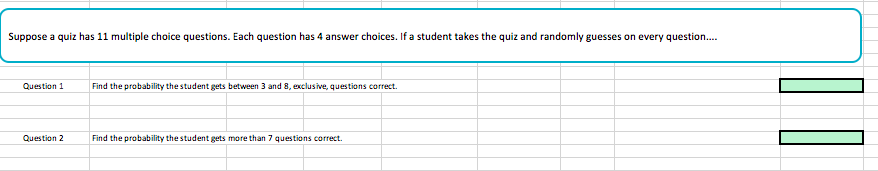Suppose a quiz has 11 multiple choice questions. Each question has 4 answer choices. If a student takes the quiz and randomly guesses on every questionQuestion 1Find the probability thestudent gets between 3 and 8, exclusive, questions correct.Question 2 Find the probability the student gets more than 7 questions correct

Questionhelp_outlineImage TranscriptioncloseSuppose a quiz has 11 multiple choice questions. Each question has 4 answer choices. If a student takes the quiz and randomly guesses on every question Question 1 Find the probability thestudent gets between 3 and 8, exclusive, questions correct. Question 2 Find the probability the student gets more than 7 questions correct fullscreen
Step 1

This question  is based on binomial probability distribution, where n = 11 and Probability of makng a correct answer is 0.25.

Since each question has only 1 correct answer  out of 4 options So probability of making correct answer is 0.25.

The formula used to get the probability is given on white board.

Step 2

We have to find the probability of getting more than 4 and less than 8 correc answers out of 11.

Step 3

Now we have to calculate the probability that more  ...

Want to see the full answer?

See Solution

Want to see this answer and more?

Our solutions are written by experts, many with advanced degrees, and available 24/7

See Solution
Tagged in

Statistics